Optics Exam4 and Problem Solutions

Optics Exam4 and  Problem Solutions

1. Find the velocity of the light in a medium having refractive index n=2.

n=c/V

2=300000/V

V=150000km/h

2. Relative refractive indexes of mediums are n1,2=2, n2,3=1,5. Find n1,3.

n1,2=n2/n1=2

n1=n2/2

n2,3=n3/n2=1,5

n3=1,5n2

n1,3=n3/n1=1,5n2/n2/2=3

3. If the ray follows following path while passing from one medium to another, find the refractive index of medium.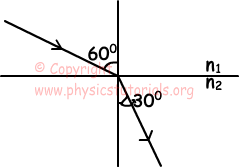n1,2=n2/n1

UsingSnell's law

n1.sin600=n2.sin300

n2/n1=sin600/sin300=√3

4.  Find the distance between point K and its image on plane mirror with respect to observer placed at point A.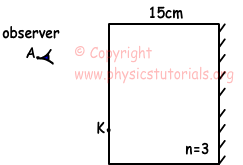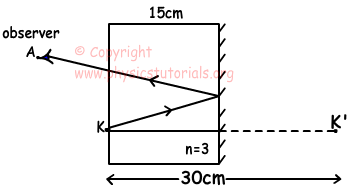happarent=nair/nmedium.hreal

happarent=1/3.30cm

happarent=10cm

5. Path of the ray S is given below on spherical medium. Find the relation between refractive indexes of these mediums.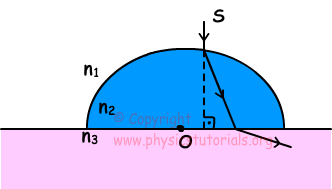As you can see from the picture given below, angles of refracted rays are larger than the angles of incident rays, Thus, relation between the refractive indexed becomes;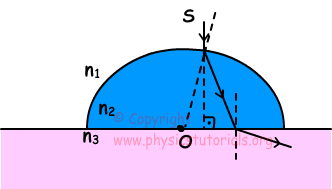n1>n2>n3

Author:

Tags: